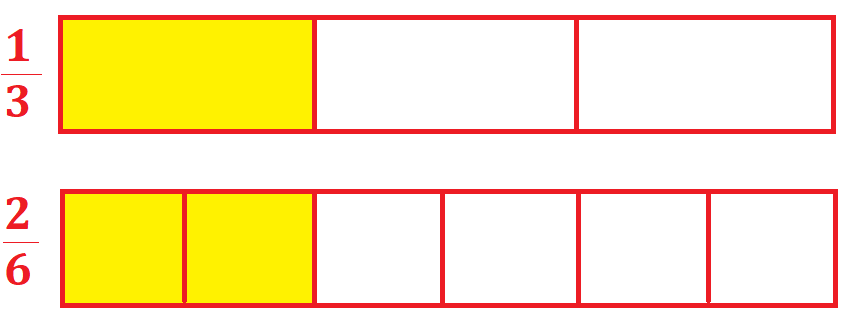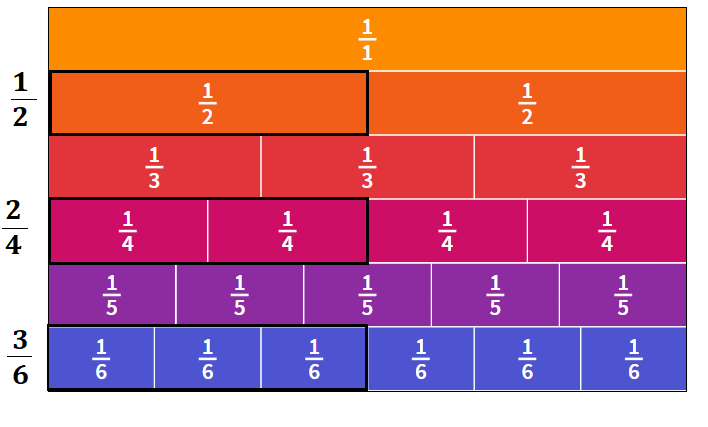# Math > Year 2

## Equivalent fractions

What is Equivalent?
Equivalent means equal in value.

What is a fraction?
A fraction is a part of a whole. A fraction has a numerator (top part) and a denominator (bottom part).

What are Equivalent Fractions?
Equivalent fractions are different fractions that have equal value.

Example of Equivalent Fractions

Using Shape:The shaded region for  is the same as the shaded region for .
Hence,   and   are equivalent fractions.

Using Bar Model:The shaded region for is the same as the shaded region for .

Hence, and  are equivalent fractions.

Using Fraction Wall://